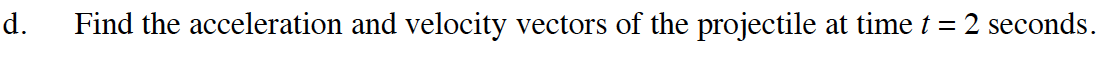### Home > CALC > Chapter 11 > Lesson 11.4.1 > Problem11-126

11-126.
1. A projectile travels through the air with position x = 15t and y = −4.9t2 + 46t + 11 where x and y are measured in meters and t is in seconds. Homework Help ✎

1. When does the projectile hit the ground?

2. How far does the projectile travel during its trip through the air? That is, find the length of the path of the projectile.

3. What is the maximum height of the projectile?

4. Find the acceleration and velocity vectors of the projectile at time t = 2 seconds.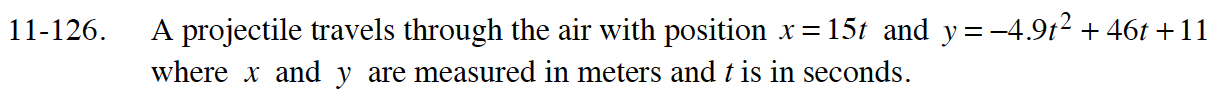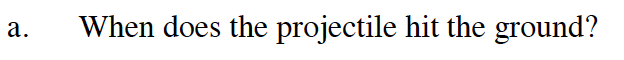When does y = 0?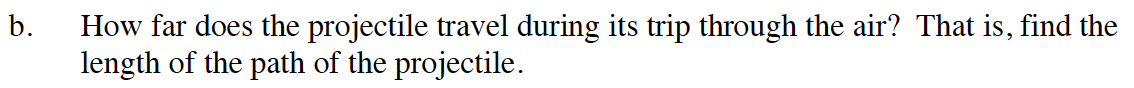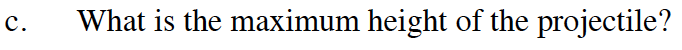This is when y′ = 0.